# Application of derivative to the study of the function

## The monotony and continuity of functions

### A sufficient condition for increasing functions

If at each point of the interval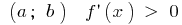, the function is increasing on this interval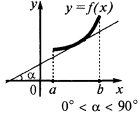### A sufficient condition for decreasing functions

If at each point of the interval, the function is decreasing on this interval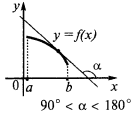Remark. These conditions are only sufficient but not necessary conditions for growth and decreasing functions

### A necessary and sufficient condition of constancy of the function

A function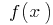is constant on an interval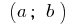if and only if when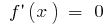all points of the interval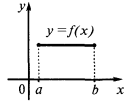## The extrema (maximums and minimums) of the function

### The maximum point

Definition: the Point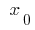of defining functionsis called a maximum point of this function if there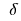is a neighborhood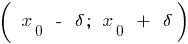of the pointthat for allfrom this neighborhood inequality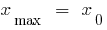— the maximum point— max

### The point of a minimum

Definition: the Pointof defining functionsis called the minimum point of this function if thereis a neighborhoodof the pointthat for allfrom this neighborhood inequality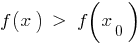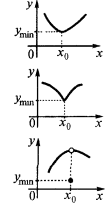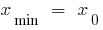— minimum point— minimum

### The critical point

Definition: the Interior points of the domain of definition of functions where the derivative is zero or does not exist are called critical

### Necessary condition for an extremum— the extremum pointornot exists

(but not at each pointwhereornot there will be an extremum!)

### Sufficient condition of extremum

at the point ofsign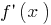changesat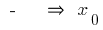point of maximum

at the point thesignchanges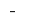at the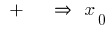point of minimum

### An example of a graph of a functionthat has an extremum— critical point## The study of the function on the monotonicity and extrema

Example.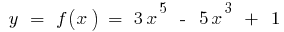1. Find the domain of definition and the intervals on which the function is continuous
2. Scope: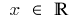The function is continuous at every point of its domain of definition

3. To find the derivative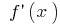4.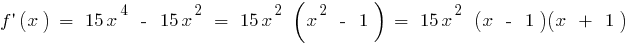5. Find the critical points, i.e. the interior points determining where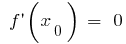or not there
6.there is in the entire scopewhen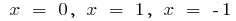7. Denote the critical point on the domain of definition, find sign of derivative and nature of function at each interval, which splits the definition area
8. For each critical point determine whether it is high or low or is not an extremum point
9.10. Record porni the result of the study (intervals of monotonicity and extrema)
11.increases withandsubsides when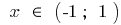Extreme points:Extremes:## Maximum and minimum values of continuous functions on the interval

Property: If the function iscontinuous on an interval and has therein a finite number of critical points, then it attains its maximum and minimum values on this interval either at a critical point belonging to this interval or at the endpoints of the interval

### Finding maximum and minimum values of continuous functions on the interval

Example.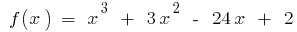when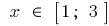1. To find the derivative2.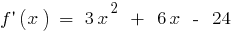3. Find the critical points (or not exists)
4.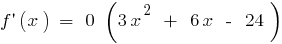ifand when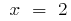5. Choose critical points that belong to a given segment
6. A given segment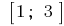belongs to only critical point7. To calculate the function values at critical points and the endpoints of the interval
8.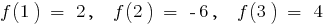9. Compare the two values and choose the smallest and largest
10.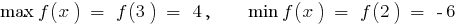Tags:
Chapter:
Versions in other languages:
Share with friends: